Display Accessibility Tools

##Prime Time: Factors and Multiples

### Factors and Multiples: Understand relationships among factors, multiples, divisors, and products.

• Classify numbers as prime, composite, even, odd, or square
• Recognize that factors of a number occur in pairs
• Recognize situations that call for common factors and situations that call for common multiples
• Recognize situations that call for the greatest common factor and situations that call for the least common multiple
• Recognize and use the fact that every whole number can be written in exactly one way as a product of prime numbers
• Use exponential notation to write repeated factors
• Relate the prime factorization of two numbers to the least common multiple and greatest common factor of two numbers
• Solve problems involving factors and multiples

### Equivalent Expressions: Understand why two expressions are equivalent.

• Relate the area of a rectangle to the Distributive Property
• Recognize that the Distributive Property relates the multiplicative and additive structures of whole numbers
• Solve problems involving the Order of Operations and Distributive Property
• Recognize that the Distributive Property relates the multiplicative and additive structures of whole numbers
• Use the properties of operations of numbers, including the Distributive Property, and the Order of Operations convention to write equivalent numerical expressions
• Solve problems involving the Order of Operations and Distributive Property

##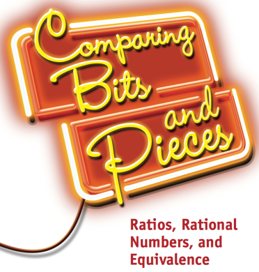Comparing Bits and Pieces: Ratios, Rational Numbers, and Equivalence

### Factors and Multiples: Understand fractions and decimals as numbers that can be located on the number line, compared, counted, partitioned, and decomposed.

• Expand interpretations of a fraction to include expressing a fraction as a part–whole relationship, as a number, and as an indicated division
• Reason about the roles of the numerator and denominator in each of the interpretations of a fraction
• Use multiple interpretations of proper fractions, improper fractions, and mixed numbers
• Use decimals to represent fractional quantities with attention to place value
• Recognize that fractions are called rational numbers and that rational numbers are points on the number line
• Use the number line to reason about rational number relationships
• Use benchmarks to estimate the values of fractions and decimals and to compare and order fractions and decimals
• Recognize that fractions can represent both locations and distances on the number line
• Recognize that a number and its opposite are at equal distances from zero on the number line; the opposite of a is –a and the opposite of –a is a
• Recognize that the absolute value of a number is its distance from 0 on the number line and use that value to describe real-world quantities
• Introduce percent as a part–whole relationship in which the whole is not necessarily out of 100, but is scaled or partitioned to be “out of 100” or “per 100”
• Apply a variety of partitioning strategies to solve problems

### Ratios as Comparisons: Understand ratios as comparisons of two numbers.

• Use ratios and associated rates to compare quantities
• Distinguish between a difference, which is an additive comparison, and a ratio, which is a multiplicative comparison
• Distinguish between fractions as numbers and ratios as comparisons
• Apply a variety of scaling strategies to solve problems involving ratios and unit rates
• Recognize that a unit rate is a ratio in which one of the quantities being compared has a value of 1; use rate language in the context of a ratio relationship
• Scale percents to predict new outcomes

### Equivalence: Understand equivalence of fractions and ratios, and use equivalence to solve problems.

• Recognize that equivalent fractions represent the same amount, distance, or location; develop strategies for finding and using equivalent fractions
• Recognize that comparing situations with different-sized wholes is difficult without some common basis of comparison
• Use partitioning and scaling strategies to generate equivalent fractions and ratios and to solve problems
• Develop meaningful strategies for representing fraction amounts greater than 1 or less than –1 as both mixed numbers and improper fractions
• Recognize that equivalent ratios represent the same relationship between two quantities; develop strategies for finding and using equivalent ratios
• Build and use rate tables of equivalent ratios to solve problems

##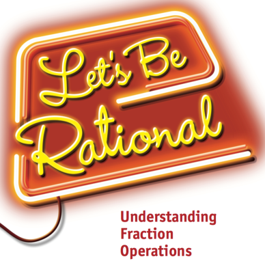Let's Be Rational: Understanding Fraction Operations

### Numeric Estimation: Understand that estimation is a tool used in a variety of situations including checking answers and making decisions, and develop strategies for estimating results of arithmetic operations.

• Use benchmarks and other strategies to estimate results of operations with fractions
• Use estimates to check the reasonableness of exact computations
• Give various reasons to estimate and identify when a situation calls for an overestimate or an underestimate
• Use estimates and exact solutions to make decisions

### Fraction Operations: Revisit and continue to develop meanings for the four arithmetic operations and skill at using algorithms for each.

• Determine when addition, subtraction, multiplication, or division is the appropriate operation to solve a problem
• Develop ways to model sums, differences, products, and quotients with areas, fraction strips, and number lines
• Use knowledge of fractions and equivalence of fractions to develop algorithms for adding, subtracting, multiplying, and dividing fractions
• Write fact families with fractions to show the inverse relationship between addition and subtraction, and between multiplication and division
• Compare and contrast dividing a whole number by a fraction to dividing a fraction by a whole number
• Recognize that when you multiply or divide a fraction, your answer might be less than or more than the numbers you started with
• Solve real-world problems using arithmetic operations on fractions

### Variables and Equations: Use variables to represent unknown values and equations to represent relationships.

• Represent unknown real-world and abstract values with variables
• Write equations (or number sentences) to represent relationships among real-world and abstract values
• Use fact families to solve for unknown values

##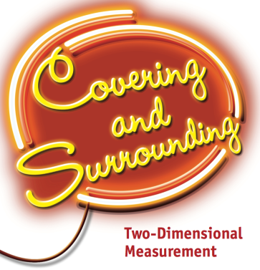Covering and Surrounding: Two-Dimensional Measurement

### Area and Perimeter

• Deepen the understanding of area and perimeter of rectangular and nonrectangular shapes
• Relate area to covering a figure
• Relate perimeter to surrounding a figure
• Analyze what it means to measure area and perimeter
• Develop and use formulas for calculating area and perimeter
• Develop techniques for estimating the area and perimeter of an irregular figure
• Explore relationships between perimeter and area, including that one can vary considerably while the other stays fixed
• Visually represent relationships between perimeter and area on a graph
• Solve problems involving area and perimeter of rectangles

### Area and Perimeter of Parallelograms and Triangles

• Analyze how the area of a triangle and the area of a parallelogram are related to each other and to the area of a rectangle
• Recognize that a triangle can be thought of as half of a rectangle whose sides are equal to the base and height of the triangle
• Recognize that a parallelogram can be decomposed into two triangles. Thus the area of a parallelogram is twice the area of a triangle with the same base and height as the parallelogram
• Know that the choice of base of a triangle (or parallelogram) is arbitrary but that the choice of the base determines the height
• Recognize that there are many triangles (or parallelograms) that can be drawn with the same base and height
• Develop formulas and strategies, stated in words or symbols, for finding the area and perimeter of triangles and parallelograms
• Find the side lengths and area of polygons on a coordinate grid
• Solve problems involving area and perimeter of parallelograms and triangles
• Solve problems involving area and perimeter of polygons by composing into rectangles or decomposing into triangles

### Surface Area of Prisms and Pyramids and Volume of Rectangular Prisms

• Extend the understanding of the volume of rectangular prisms
• Relate volume to filling a three-dimensional figure
• Extend understanding of the strategies for finding the volume of rectangular prisms to accommodate fractional side lengths
• Relate finding area of two-dimensional shapes to finding the surface area of three-dimensional objects
• Develop strategies for finding the surface area of three-dimensional objects made from rectangles and triangles
• Solve problems involving surface area of prisms and pyramids and volume of rectangular prisms

##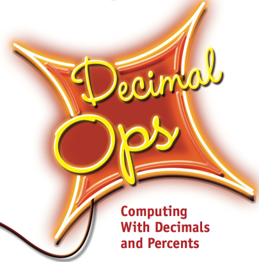Decimal Ops: Computing with Decimals and Percents

### Numeric Estimation: Understand estimation as a tool for a variety of situations, including checking answers and making decisions.

• Use estimates to compute products and to check all four operations

### Decimal Operations: Revisit and develop meanings for the four arithmetic operations on whole numbers and decimals, and skill at using algorithms for each decimal operation.

• Recognize when addition, subtraction, multiplication or division is the appropriate operation to solve a problem
• Use place value to relate operations with decimals to the same operations with fractions, and to develop understanding of algorithms
• Extend understanding of multiplication and division of multi-digit whole numbers and develop standard algorithms for multiplying and dividing decimals with the aid of at most paper and pencil
• Find a repeating or terminating decimal equivalent to a given rational number
• Solve problems using arithmetic operations on decimals, including finding unit rates

### Variables and Equations: Use variables to represent unknown values and equations to represent relationships.

• Write equations or number sentences to represent relationships among real-world and abstract values
• Use fact families to write and solve equivalent sentences
• Use multiplication sentences to check division sentences

### Percents: Develop understanding of various contexts in which percentages are used, including sales tax, tips, discounts, percent increases.

• Connect ratios and unit rates to percentages
• Develop ways to model percent problems
• Write and solve a number sentence to find a percent of a given amount, to find the total amount given the percent amount and rate, to find the original amount given the percent rate of increase/decrease and the amount of the original +/– the increase/decrease, and to find the percent that one number is of another

##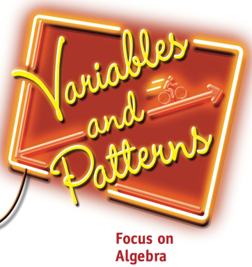Variables and Patterns: Focus on Algebra

### Variables and Patterns (Relationships): Develop understanding of variables and how they are related.

• Explore problem situations that involve variables and relationships
• Identify the dependent and independent variable and describe how they are related in a situation
• Interpret the ‘stories’ told by patterns in tables and coordinate graphs of numeric (x, y) data;
• Represent the pattern of change between two variables in words, data tables, graphs, and equations
• Investigate situations that change over time
• Examine increasing and decreasing patterns of change
• Compare linear and non-linear patterns of change by using tables or graphs
• Use tables, graphs and equations to find the value of a variable given the value of the associated variable
• Explore relationships to become familiar with graphing in all in four quadrants
• Describe advantages and disadvantages of using words, tables, graphs and equations to represent patterns of change between two variables and make connections across those representations
• Write an equation to express the relationship between two variables in one and two operations: y = mx, y = b + x, and y = b + mx
• Calculate average speed and show how it is reflected in a table or graph and vice versa.
• Recognize and express direct proportionality relationships with a unit rate (y = mx) and represent these relationships in rate tables and graphs
• Solve problems that involve variables

### Expressions and Equations: Develop understanding of expressions and equations.

• Use properties of operations, including the Distributive Property and the Order of Operations, to write equivalent expressions for the dependent variable in terms of the independent variable
• Use tables, graph or properties of numbers such as the Distributive Property to show that two expressions are equivalent
• Identify parts of an expression using mathematical terms (sum, term, product, factor, quotient, coefficient); view one or more parts of an expression as a single entity
• Interpret and evaluate expressions in which letters stand for numbers and apply the Order of Operations as needed
• Recognize that equations are statements of equivalence between two expressions
• Solve linear equations of the form, y = ax, y = b + x, and y = b + ax using numeric guess and check, tables of (x, y) values, graphs or fact families
• Write an inequality and associate it with an equation to find solutions and graph the solutions on a number line

##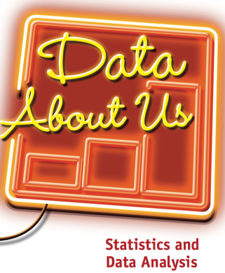Data About Us: Statistics and Data Analysis

### Understand and use the process of statistical investigation: pose questions, collect and analyze data, and make interpretations to answer questions.

• Apply Guidelines for Describing Distributions as a tool to be used with the analyzing and interpreting phases of the statistical investigation process
• Construct and use simple surveys as a method of collecting data

### Analyze data distributions

• Distinguish data and data types
• Recognize data consist of counts or measurements of a variable that are called a distribution of data values
• Distinguish between categorical data and numerical data and identify which graphs and statistics may be used to represent each kind of data
• Use multiple representations
• Organize and represent data using tables, dot plots, line plots, value bar graphs, frequency bar graphs, histograms, and box-and-whisker plots
• Make informed decisions about which graphs/tables are used to display data being analyzed (ties back to questions asked, data types, etc.)
• Recognize that data displayed using a graph shows the overall shape of a distribution and gives a general sense of whether the data values are or are not symmetrical around a central value or if there is something unusual about the shape
• Recognize that a single number may be computed and used to characterize the center or what’s typical for a distribution of data
• Distinguish and compute measures of central tendency: the mean, median, or mode of the data
• Identify how the median and mean respond to changes in the number and magnitude of data values in a distribution
• Make informed decisions about which measures of central tendency (mean, median, or mode) may be used to describe a data distribution
• Recognize that variability occurs whenever data are collected and describe the variability in the distribution of a given data set
• Describe the amount of variability in a distribution, noting if the data values are pretty much the same or are quite spread out
• Distinguish and compute measures of spread: range, interquartile range (IQR), and mean absolute deviation (MAD)
• Develop strategies for analyzing and/or comparing data distributions
• Identify which statistical measures of center and spread (mean, median, mode, range, etc.) are most appropriate to use to describe a distribution of data
• Use measures of center and spread to compare data distributions